Courses

# RD Sharma Solutions - 17.4, Constructions, Class 7, Math Class 7 Notes | EduRev

## Class 7 : RD Sharma Solutions - 17.4, Constructions, Class 7, Math Class 7 Notes | EduRev

The document RD Sharma Solutions - 17.4, Constructions, Class 7, Math Class 7 Notes | EduRev is a part of the Class 7 Course RD Sharma Solutions for Class 7 Mathematics.
All you need of Class 7 at this link: Class 7

#### Question 1:

Construct ∆ ABC in which BC = 4 cm, ∠B = 50° and ∠C = 70°.

Steps of construction:

1. Draw a line segment BC of length 4 cm.
2. Draw ∠CBX such that ∠CBX=50°.
3. Draw ∠BCY with Y on the same side of BC as X such that ∠BCY = 70°°.
4. Let CY and BX intersect at A.
5. ABC is the required triangle.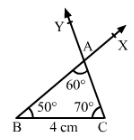#### Question 2:

Draw ∆ ABC in which BC = 8 cm, ∠B = 50° and ∠A = 50°.

∠ABC+∠BCA+∠CAB=180°
∠BCA=180°−∠ABC−∠CAB
∠BCA=180°−100°=80°

Steps of construction:

1. Draw a line segment BC of length 8 cm.
2. Draw ∠CBX such that ∠CBX = 50°.
3. Draw ∠BCY with Y on the same side of BC as X such that ∠BCY = 80°°.
4. Let CY and BX intersect at A.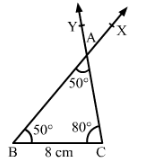#### Question 3:

Draw ∆ PQR in which ∠Q = 80°, ∠R = 55° and QR = 4.5 cm. Draw the perpendicular bisector of side QR.

Steps of construction:

1. Draw a line segment QR = 4.5 cm.
2. Draw ∠RQX = 80° and ∠QRY =55°.
3. Let QX and RY intersect at P so that PQR is the required triangle.
4. With Q as centre and radius more that 2.25 cm, draw arcs on either sides of QR.
5. With R as centre and radius more than 2.25 cm, draw arcs intersecting the previous arcs at M and N.
6. Join MN; MN is the required perpendicular bisector of QR.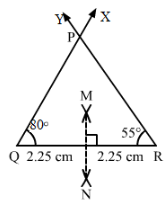#### Question 4:

Construct ∆ ABC in which AB = 6.4 cm, ∠A = 45° and ∠B = 60°.

Steps of construction:

1. Draw a line segment AB = 6.4 cm.
2. Draw ∠BAX = 45°∠BAX = 45°.
3. Draw ∠ABY with Y on the same side of AB as X such that ∠ABY = 60°°.

Let AX and BY intersect at C; ABC is the required triangle.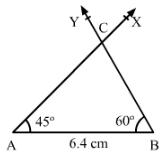#### Question 5:

Draw ∆ ABC in which AC = 6 cm, ∠A = 90° and ∠B = 60°.

We can see that ∠A+∠B+∠C = 180°. Therefore ∠C = 180°° − 60°° − 90°° = 30°.

Steps of construction:

1. Draw a line segment AC = 6 cm.
2. Draw ∠ACX=30°.
3. Draw ∠CAY with Y on the same side of AC as X such that ∠CAY = 90°°.
4. Join CX and AY. Let these intersect at B.
5. ABC is the required triangle where angle ∠ABC = 60°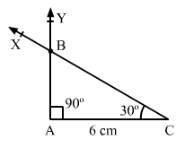Offer running on EduRev: Apply code STAYHOME200 to get INR 200 off on our premium plan EduRev Infinity!

97 docs

,

,

,

,

,

,

,

,

,

,

,

,

,

,

,

,

,

,

,

,

,

,

,

,

,

,

,

,

,

,

;